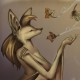### Re: Need help creating a design similar with the picturesRD
Revar Desmera
Tue, May 10, 2022 2:23 AM

I’ve been working on the BOSL2 library for a while, so my first instinct on how to implement it is probably not immediately obvious.  I’ve implemented it using `vnf_vertex_array()`. The code below has the benefit of rounding over the transition between flat and sloped using `arc()` in the profile, so I figured I’d post it.  You can tweak the profile path to arrange different flat-slope-flat variations:

start_ang = 180;
trans_ang = 270;
end_ang = 360;
major_r = 50;
base_z = 5;
top_z = 25;
width = 20;
groove_r = 5;
arc_ang = 120;
trans_r = 30;

function path_at_z(a, z) = zrot(a, p=xrot(90, p=path3d([
[major_r-width/2,0], [major_r-width/2,z],
each arc(r=groove_r, cp=[major_r,z+groove_r*cos(arc_ang/2)], start=270-arc_ang/2, angle=arc_ang),
[major_r+width/2,z], [major_r+width/2,0],
])));
profile = [ // angle x z_height
[start_ang,top_z],
each arc(n=10, r=trans_r, corner=[[start_ang,top_z], [trans_ang,top_z], [end_ang,base_z]]),
[end_ang,base_z]
];
vnf = vnf_vertex_array([
for (a = [start_ang:2:end_ang])
path_at_z(a, lookup(a,profile))
], caps=true, col_wrap=true);
vnf_polyhedron(vnf, convexity=8);

• Revar

On May 8, 2022, at 5:31 PM, sorin vatasoiu via Discuss discuss@lists.openscad.org wrote:

From: sorin vatasoiu svatasoiu@yahoo.com
Subject: [OpenSCAD] Need help creating a design similar with the pictures
Date: May 8, 2022 at 5:31:07 PM PDT

Hi,

I'm trying to simulate this knob. I can handle everything but the circular side with a channel on it.

I can do the flat circular side with the channel, but I cannot figure out how to do the halt side that has an inclination.
the code I have for the side part it is:

\$fn = 360;

outsideChannelFlat();
//channelProfile();

module outsideChannelFlat(){
rotate_extrude(angle = 180, convexity = 2) {
translate([57.5/2,0,0])
channelProfile();
}
}

module channelProfile(){
difference(){
square([7.2,16.5],center = false);
translate([7.2/2,16.5,0])
circle(\$fn = 180,  r = 4.5/2);
}
}

Any help with the inclined part it is welcomed.

Thank you,

Sorin

<sideChannel_1.JPG><IMG_20220508_163742673_1.JPG><IMG_20220508_163749087_1.JPG><IMG_20220508_163718417_1.jpg>